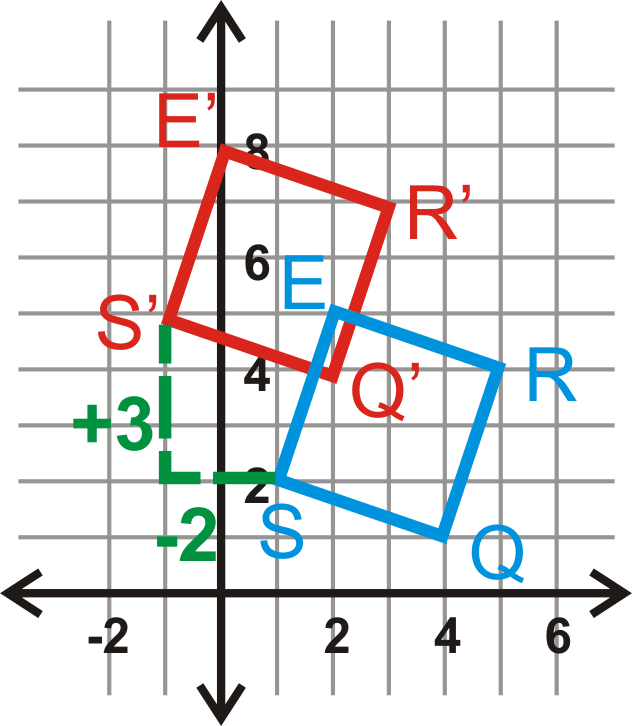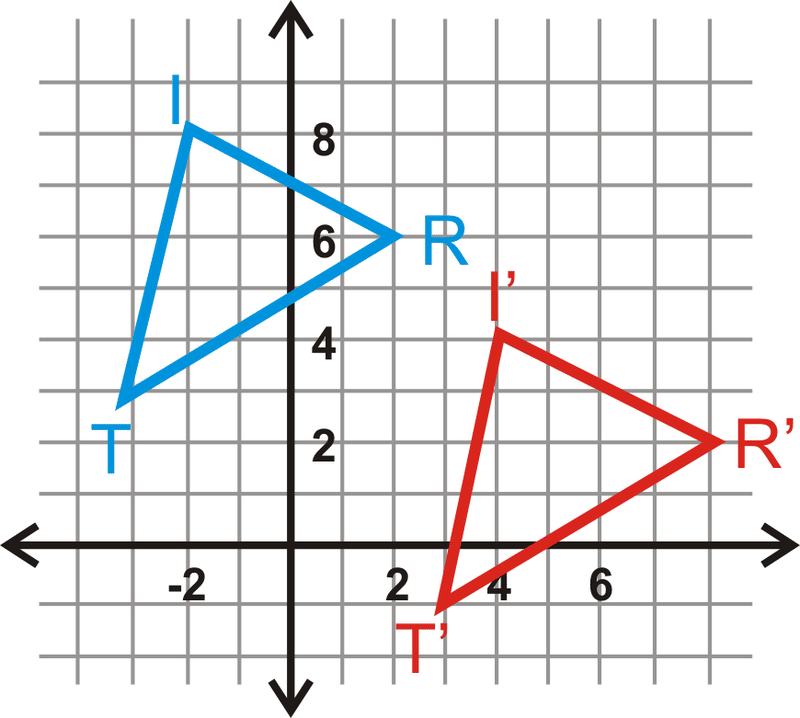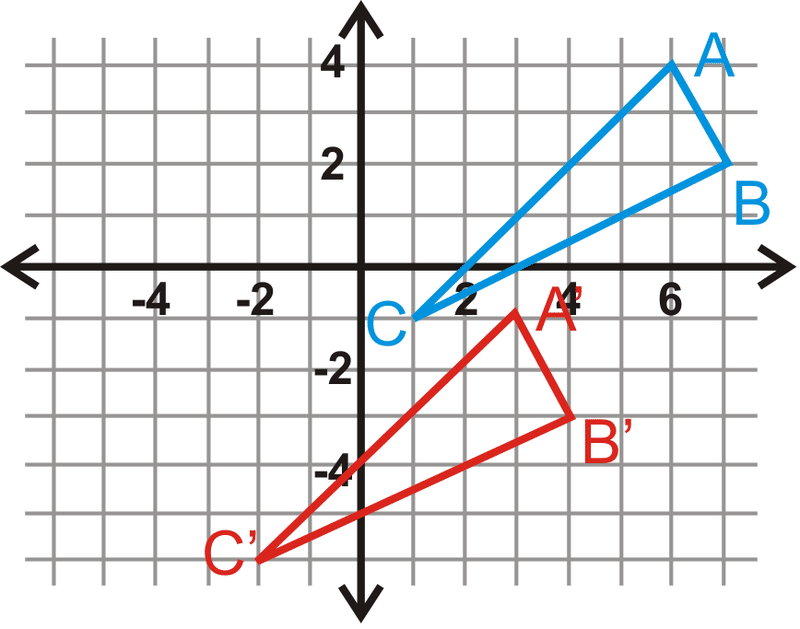# 8.5: Geometric Translation

•• Contributed by CK12
• CK12

Understand translations as movement of every point in a figure the same distance in the same direction. Graph images given preimage and translation.

## Translations

A transformation is an operation that moves, flips, or otherwise changes a figure to create a new figure. A rigid transformation (also known as an isometry or congruence transformation) is a transformation that does not change the size or shape of a figure.

The rigid transformations are translations, reflections, and rotations. The new figure created by a transformation is called the image. The original figure is called the preimage. If the preimage is $$A$$, then the image would be $$A′$$, said “a prime.” If there is an image of $$A′$$, that would be labeled $$A′′$$, said “a double prime.”

A translation is a transformation that moves every point in a figure the same distance in the same direction. For example, this transformation moves the parallelogram to the right 5 units and up 3 units. It is written $$(x,y)\rightarrow (x+5, y+3)$$.Figure $$\PageIndex{1}$$

What if you were given the coordinates of a quadrilateral and you were asked to move that quadrilateral 3 units to the left and 2 units down? What would its new coordinates be?

Example $$\PageIndex{1}$$

Triangle $$\Delta ABC$$ has coordinates $$A(3,−1)$$, $$B(7,−5)$$ and $$C(−2,−2)$$. Translate $$\Delta ABC$$ to the left 4 units and up 5 units. Determine the coordinates of $$\Delta A′B′C′$$.Figure $$\PageIndex{2}$$

Solution

Graph $$\Delta ABC$$. To translate $$\Delta ABC$$, subtract 4 from each $$x$$ value and add 5 to each $$y$$ value of its coordinates.

\begin{aligned} &A(3,−1)\rightarrow (3−4,−1+5)=A′(−1,4) \\ &B(7,−5)\rightarrow (7−4,−5+5)=B′(3,0) \\ &C(−2,−2)\rightarrow (−2−4,−2+5)=C′(−6,3) \end{aligned}

The rule would be $$(x,y)\rightarrow (x−4, y+5)$$.

Example $$\PageIndex{2}$$

Using the translation $$(x,y)\rightarrow (x+2, y−5)$$, what is the image of $$A(−6, 3)$$?

Solution

$$A′(−4,−2)$$

Example $$\PageIndex{3}$$

Graph square $$S(1,2)$$, $$Q(4,1)$$, $$R(5,4)$$ and $$E(2,5)$$. Find the image after the translation $$(x,y)\rightarrow (x−2,y+3)$$. Then, graph and label the image.

Solution

We are going to move the square to the left 2 and up 3.Figure $$\PageIndex{3}$$

\begin{aligned}(x,y)&\rightarrow (x−2,y+3) \\ S(1,2)&\rightarrow S′(−1,5) \\ Q(4,1)&\rightarrow Q′(2,4) \\ R(5,4)&\rightarrow R′(3,7) \\ E(2,5)&\rightarrow E′(0,8)\end{aligned}

Example $$\PageIndex{4}$$

Find the translation rule for $$\Delta TRI$$ to $$\Delta T′R′I′$$.

Solution

Look at the movement from $$T$$ to $$T′$$. The translation rule is $$(x,y)\rightarrow (x+6, y−4)$$.Figure $$\PageIndex{4}$$Figure $$\PageIndex{5}$$

## Review

Use the translation $$(x,y)\rightarrow (x+5, y−9)$$ for questions 1-7.

1. What is the image of $$A(−1,3)$$?
2. What is the image of $$B(2,5)$$?
3. What is the image of $$C(4,−2)$$?
4. What is the image of $$A′$$?
5. What is the preimage of $$D′(12,7)$$?
6. What is the image of $$A′′$$?
7. Plot $$A$$, $$A′$$, $$A′′$$, and $$A′′′$$ from the questions above. What do you notice?

The vertices of $$\Delta ABC$$ are $$A(−6,−7)$$, $$B(−3,−10)$$ and $$C(−5,2)$$. Find the vertices of $$\Delta A′B′C′$$, given the translation rules below.

1. $$(x,y)\rightarrow (x−2, y−7)$$
2. $$(x,y)\rightarrow (x+11, y+4)$$
3. $$(x,y)\rightarrow (x, y−3)$$
4. $$(x,y)\rightarrow (x−5, y+8)$$
5. $$(x,y)\rightarrow (x+1, y)$$
6. $$(x,y)\rightarrow (x+3, y+10)$$

In questions 14-17, $$\Delta A′B′C′$$ is the image of $$\Delta ABC$$. Write the translation rule.

1.Figure $$\PageIndex{6}$$
2.Figure $$\PageIndex{7}$$
3.Figure $$\PageIndex{8}$$
4.Figure $$\PageIndex{9}$$

Use the triangles from #17 to answer questions 18-20.

1. Find the lengths of all the sides of $$\Delta ABC$$.
2. Find the lengths of all the sides of $$\Delta A′B′C′$$.
3. What can you say about $$\Delta ABC$$ and $$\Delta A′B′C′$$? Can you say this for any translation?
4. If $$\Delta A′B′C′$$ was the preimage and $$\Delta ABC$$ was the image, write the translation rule for #14.
5. If $$\Delta A′B′C′$$ was the preimage and $$\Delta ABC$$ was the image, write the translation rule for #15.
6. Find the translation rule that would move $$A$$ to $$A′(0,0)$$, for #16.
7. The coordinates of $$\Delta DEF$$ are $$D(4,−2)$$, $$E(7,−4)$$ and $$F(5,3)$$. Translate $$\Delta DEF$$ to the right 5 units and up 11 units. Write the translation rule.
8. The coordinates of quadrilateral $$QUAD$$ are $$Q(−6,1)$$, $$U(−3,7)$$, $$A(4,−2)$$ and $$D(1,−8)$$. Translate $$QUAD$$ to the left 3 units and down 7 units. Write the translation rule.